Courses

# Test: Basic Geometrical Ideas - 3

## 20 Questions MCQ Test Mathematics (Maths) Class 6 | Test: Basic Geometrical Ideas - 3

Description
This mock test of Test: Basic Geometrical Ideas - 3 for Class 6 helps you for every Class 6 entrance exam. This contains 20 Multiple Choice Questions for Class 6 Test: Basic Geometrical Ideas - 3 (mcq) to study with solutions a complete question bank. The solved questions answers in this Test: Basic Geometrical Ideas - 3 quiz give you a good mix of easy questions and tough questions. Class 6 students definitely take this Test: Basic Geometrical Ideas - 3 exercise for a better result in the exam. You can find other Test: Basic Geometrical Ideas - 3 extra questions, long questions & short questions for Class 6 on EduRev as well by searching above.
QUESTION: 1

Solution:
QUESTION: 2

Solution:
QUESTION: 3

### Name the set of points which is a part of a line with two end points.

Solution:
QUESTION: 4

How is a line PQ symbolically written?

Solution:

The symbol of a line is ↔.
Hence, a line PQ is symbolically written as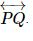QUESTION: 5

How do you write a line segment AB symbolically?

Solution:
QUESTION: 6

What is the symbolic representation of a ray OP?

Solution:
QUESTION: 7

What are used to represent points?

Solution:
QUESTION: 8

What is the number of end points of a line?

Solution:
QUESTION: 9

What is a set of points extending infinitely in all directions on the same flat surface called?

Solution:
QUESTION: 10

How many lines can be drawn passing through a given point?

Solution:
QUESTION: 11

How many lines can you draw joining two distinct points in a plane?

Solution:

Only one line can be drawn passing through two given points.QUESTION: 12

Three points P, Q and R are said to be collinear. Where do they lie?

Solution: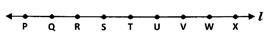The points P, Q, R, S, T ... lying on line ℓ are said to be collinear.
NOTE: Infinite number of points lying on same line are considered as collinear.

QUESTION: 13

Four lines p, q, r and s are said to be concurrent. What do they pass through?

Solution:

If the lines p, q, r and s are said to be concurrent they pass through the same point.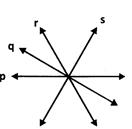QUESTION: 14

In the given figure what is point P called?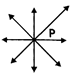Solution:
QUESTION: 15

In the given figure, what are lines l, m and n called?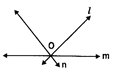Solution:
QUESTION: 16

In the given figure, what are points P, O and Q called?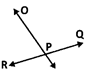Solution:
QUESTION: 17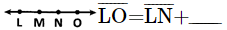Solution:
QUESTION: 18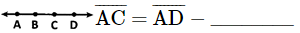Solution:
QUESTION: 19

Which instrument is used to compare two line segments?

Solution:

A divider is used to compare line segments.

QUESTION: 20

Which of these instruments are used to construct a line segment?

Solution: